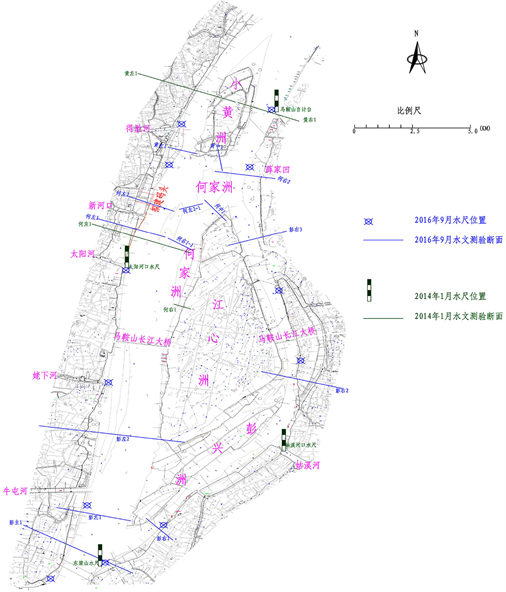#### 期刊菜单

Analysis of Flood Control Impact of Hexian Wharf Project
DOI: 10.12677/HJCE.2022.116088, PDF, HTML, XML, 下载: 84  浏览: 125  国家自然科学基金支持

Abstract: In order to implement the spirit of General Secretary Xi Jinping’s important speech on further promoting the development of the Yangtze River Economic Belt, improving the utilization efficiency of the Yangtze River port coastline, and promoting the high-quality development of the Yangtze River port and the economy and society, it is proposed to construct the Wanjiang bulk sand and gravel intermodal transport center wharf project. Through the calculation and analysis of two-dimensional numerical model, the influence of proposed wharf on river regime evolution and river flood is demonstrated. The results show that after the completion of the wharf, the influence on the river regime and flood control of the engineering reach is small. The river regime evolution of the proposed project office in recent years is mainly characterized by left scouring and right silting, and the water depth of the left bank is good, which is suitable for wharf construction.

1. 工程概况

1.1. 拟建码头基本情况

1.2. 河道概况

1.3. 河势演变

2. 模型建立与验证

2.1. 平面二维数学模型基本原理

2.1.1. 数学模型

$\frac{\partial H}{\partial t}+\frac{1}{{C}_{\xi }{C}_{\eta }}\frac{\partial }{\partial \xi }\left(hu{C}_{\eta }\right)+\frac{1}{{C}_{\xi }{C}_{\eta }}\frac{\partial }{\partial \eta }\left(hv{C}_{\xi }\right)=0$ (1)

$\xi$ 方向动量方程

$\begin{array}{l}\frac{\partial u}{\partial t}+\frac{1}{{C}_{\xi }{C}_{\eta }}\left[\frac{\partial }{\partial \xi }\left({C}_{\eta }{u}^{2}\right)+\frac{\partial }{\partial \eta }\left({C}_{\xi }vu\right)+vu\frac{\partial {C}_{\eta }}{\partial \eta }-{v}^{2}\frac{\partial {C}_{\eta }}{\partial \xi }\right]\\ =-g\frac{1}{{C}_{\xi }}\frac{\partial H}{\partial \xi }+fv-\frac{u\sqrt{{u}^{2}+{v}^{2}}{n}^{2}g}{{h}^{4/3}}+\frac{1}{{C}_{\xi }{C}_{\eta }}\left[\frac{\partial }{\partial \xi }\left({C}_{\eta }{\sigma }_{\xi \xi }\right)+\frac{\partial }{\partial \eta }\left({C}_{\xi }{\sigma }_{\eta \xi }\right)+{\sigma }_{\xi \eta }\frac{\partial {C}_{\xi }}{\partial \eta }-{\sigma }_{\eta \eta }\frac{\partial {C}_{\eta }}{\partial \xi }\right]\end{array}$ (2)

$\eta$ 方向动量方程

$\begin{array}{l}\frac{\partial v}{\partial t}+\frac{1}{{C}_{\xi }{C}_{\eta }}\left[\frac{\partial }{\partial \xi }\left({C}_{\eta }vu\right)+\frac{\partial }{\partial \eta }\left({C}_{\xi }{v}^{2}\right)+uv\frac{\partial {C}_{\eta }}{\partial \xi }-{u}^{2}\frac{\partial {C}_{\xi }}{\partial \eta }\right]\\ =-g\frac{1}{{C}_{\eta }}\frac{\partial H}{\partial \eta }-fu-\frac{v\sqrt{{u}^{2}+{v}^{2}}{n}^{2}g}{{h}^{4/3}}+\frac{1}{{C}_{\xi }{C}_{\eta }}\left[\frac{\partial }{\partial \xi }\left({C}_{\eta }{\sigma }_{\xi \eta }\right)+\frac{\partial }{\partial \eta }\left({C}_{\xi }{\sigma }_{\eta \eta }\right)+{\sigma }_{\eta \xi }\frac{\partial {C}_{\eta }}{\partial \xi }-{\sigma }_{\xi \xi }\frac{\partial {C}_{\xi }}{\partial \eta }\right]\end{array}$ (3)

${C}_{\xi }=\sqrt{{x}_{\xi }^{2}+{y}_{\xi }^{2}},\text{}{C}_{\eta }=\sqrt{{x}_{\eta }^{2}+{y}_{\eta }^{2}}$

${\sigma }_{\xi \xi }$${\sigma }_{\xi \eta }$${\sigma }_{\eta \xi }$${\sigma }_{\eta \eta }$ 表示紊动应力：

$\begin{array}{l}{\sigma }_{\xi \xi }=2{\upsilon }_{t}\left[\frac{1}{{C}_{\xi }}\frac{\partial u}{\partial \xi }+\frac{v}{{C}_{\xi }{C}_{\eta }}\frac{\partial {C}_{\xi }}{\partial \eta }\right],\text{}{\sigma }_{\eta \eta }=2{\upsilon }_{t}\left[\frac{1}{{C}_{\eta }}\frac{\partial v}{\partial \eta }+\frac{u}{{C}_{\xi }{C}_{\eta }}\frac{\partial {C}_{\eta }}{\partial \xi }\right]\\ \text{}{\sigma }_{\xi \eta }={\sigma }_{\eta \xi }={\upsilon }_{t}\left[\frac{{C}_{\eta }}{{C}_{\xi }}\frac{\partial }{\partial \xi }\left(\frac{v}{{C}_{\eta }}\right)+\frac{{C}_{\xi }}{{C}_{\eta }}\frac{\partial }{\partial \eta }\left(\frac{u}{{C}_{\xi }}\right)\right]\end{array}$

${\nu }_{t}$ 表示紊动粘性系数。

2.1.2. 数值解法

${C}_{\xi }{C}_{\eta }\frac{\partial \psi }{\partial t}+\frac{\partial \left({C}_{\eta }u\psi \right)}{\partial \xi }+\frac{\partial \left({C}_{\xi }v\psi \right)}{\partial \eta }=\frac{\partial }{\partial \xi }\left(\Gamma \frac{{C}_{\eta }}{{C}_{\xi }}\frac{\partial \psi }{\partial \xi }\right)+\frac{\partial }{\partial \eta }\left(\Gamma \frac{{C}_{\xi }}{{C}_{\eta }}\frac{\partial \psi }{\partial \eta }\right)+C$ (4)

2.2. 计算区域及网格划分

2.3. 计算工况及概化

2.3.1. 计算工况Table 1. Model calculation conditions

2.3.2. 工程概化

① 由于工程桩基的影响，增加了过水湿周，从而引起阻力的增加。假定单元内流速分布均匀、摩阻比降相同，用以下公式对局部糙率 ${n}_{p}$ 进行修正：

${n}_{p}=\alpha {n}_{2}{\left[1+2{\left(\frac{{n}_{1}}{{n}_{2}}\right)}^{2}\frac{h}{B}\right]}^{0.5}$

② 在防洪设计洪水条件下，码头梁板将被淹没。将梁板的阻水部分按断面突然缩小的建筑物考虑，并通过下式换算得到相应的附加糙率系数。

${n}_{f}={h}^{1/6}\sqrt{\frac{\zeta }{8g}}$

③ 总糙率系数为修正后的局部糙率系数np和附加糙率系数nf的几何平均。加糙后工程区总糙率系数在0.05~0.09之间。

2.4. 数学模型建立与验证

1) 基本资料

① 地形资料

② 水流运动观测资料

2) 率定与验证计算成果

a) 水位验证

3. 拟建工程对河势影响

3.1. 河势演变特点

3.2. 河势演变分析Figure 1. Distribution map of hydrological testTable 2. Water level verification form (January 2014) Unit (m)

4. 拟建工程对防洪的影响分析

4.1. 对河道行洪断面的影响

4.2. 对河道行洪安全产生的影响

5. 结论

1) 工程区域范围内可能导致河床一定的冲淤变化，但范围有限，整个河段河势不会因码头工程建设而发生较大范围的变化。港池岸坡加强护砌后，进一步确保了河势稳定。

2) 工程设计洪水(10.45 m)条件下，阻水面积400.2 m2，占整个河道行洪断面面积(64,243 m2)的0.62%，河道水位、流速场及局部流场影响范围集中在工程局部区域内，对河道行洪影响较小。

3) 引桥近堤桥墩缩短了堤基渗透路径、接堤处车辆利用短增加了堤身荷载等对堤防渗流及抗滑安全不利，应采取堤防加固、局部防渗、堤坡护砌等补救措施。

  郝婕妤, 方娟娟, 李会云, 杨光荣. 长江马鞍山河段洲滩演变特征及影响因素分析[J]. 水利水电快报, 2020, 41(2): 17-21+39. https://doi.org/10.15974/j.cnki.slsdkb.2020.02.004  刘星童, 渠庚, 徐一民. 长江马鞍山河段演变规律与治理思路研究[J]. 人民长江, 2021, 52(6): 1-6. https://doi.org/10.16232/j.cnki.1001-4179.2021.06.001  刘星童. 水沙变化下长江马鞍山河段河道演变及治理方案研究[D]: [硕士学位论文]. 昆明: 昆明理工大学, 2021. https://doi.org/10.27200/d.cnki.gkmlu.2021.000998  孙业山. 长江马鞍山段河道演变及治理方案探讨[J]. 江淮水利科技, 2019(6): 32-33.  唐金武, 由星莹, 侯卫国, 王罗斌. 长江下游马鞍山河段演变趋势分析[J]. 泥沙研究, 2015(1): 30-35. https://doi.org/10.16239/j.cnki.0468-155x.2015.01.006